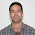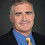## Friday, September 13, 2002

### Complexity Class of the Week: Factoring

Previous CCW

Factoring is not a class, it is not even a language. Nevertheless it has some interesting connections to complexity classes that are worth discussing.

Factoring is the problem of taking a number m and producing the prime factors of m. There are a number of algorithms for factoring but they all take time exponential in the length of the input (log m) in the worst case. Here is a good place to start reading about these algorithms.

Factoring gives a great example of an issue that often arises in complexity, search versus decision. It has always amazed me that we can easily determine whether a number has nontrivial factors but finding these factors is apparently much more difficult. This distinction and some other nice properties of numbers makes factoring very useful in cryptography.

To consider the computational complexity of factoring, we need to make a language version.

FACTOR = {(m,r) | There is an s, 1<s<r such that s divides m}

FACTOR is computationally equivalent to factoring: (m,r) is in FACTOR if and only if r is greater than the least prime factor of m. Given a black-box for FACTOR one can use binary search to find a prime factor of m.

If one insists on a complexity class, one could consider all the languages reducible to FACTOR but there is not much value beyond considering the complexity of the language FACTOR.

FACTOR is in NP∩co-NP: Guess the prime factorization of m. Since every number has a unique prime factorization we have FACTOR in UP∩co-UP where UP is the set of languages accepted by NP machines which have at most one accepting path for every input. The class UP∩co-UP is arguably the smallest interesting complexity class not known to have efficient algorithms and, assuming factoring is hard, really does not have efficient algorithms. I should note that FACTOR in UP∩co-UP was known before the recent AKS primality algorithm but the proof was more difficult.

Peter Shor almost singlehandedly justified the study of quantum computing by his efficient quantum algorithm for factoring, in complexity terms FACTOR in BQP. His proof used the fact that factoring of a number m can be reduced to finding the order of the multiplicative group (mod n). Shor then shows that quantum computers can solve this kind of group question.

Factoring does not appear to be hard for any nontrivial complexity class. This means that unlike Satisfiability we don't have any reason to believe that factoring is hard other than the fact that many smart people have failed to solve it. On the other hand the hardness of factoring is taken as a given in the study of complexity and cryptography and used to show the hardness of classes like UP∩co-UP.

1.Hi there,

I share a least two common facts with Pierre de Fermat, I am French and I am an amateur mathematician mostly working on number theory.
I have been to many of your interesting posts in this blog and I guess you are highly qualified to answer my simple questions. Two questions exactly :

1. Apart from the cryptography catastrophe, would there be some benefits of factoring being as fast as multiplication ? Any other practical aspects ?

2. What would You Do, personally if E.T. would give you a simple ultra fast factoring algorithm ?

Eric Pouhier

2.Hello Dr. Fortnow,

I have a doubt in the class UP in which you surely could help me. I used the definition that I found in wikipedia about the UP class:

if x in L , then there exists a unique certificate y with |y| = O(|x|c) such that A(x,y) = 1
if x isn't in L, there is no certificate y with |y| = O(|x|c) such that A(x,y) = 1

I thought this definition was correct, even though wikipedia might have had errors in this topic. However, I used this definition on an informal presentation of this topic and this caused confusion with the US class, even though I refered all the time in terms of unique certifcate.

Could you tell me if this definition is wrong, please?

I would appreciate your help, because I really need it.

1.Yes, your definition is correct. US does can allow 0, 2 or more accepting assignments if x is not in L.

2.Thank you very much. Sometimes, it's better not to trust 100% percent in ourselves(the people who are not excellent scientists yet such as students of any kind or followers of this issue in general), because we don't have to know everything and we might be wrong in many cases, for that reason it's very good to have the chance of receive an answer of an expert like you. Thanks again.

3.Why is factoring in UP\cap coUP? Can you elaborate? There are many factors (witnesses).